# A high performance test of differential gene expression for oligonucleotide arrays

## Abstract

Logit-t employs a logit-transformation for normalization followed by statistical testing at the probe-level. Using four publicly-available datasets, together providing 2,710 known positive incidences of differential expression and 2,913,813 known negative incidences, performance of statistical tests were: Logit-t provided 75% positive-predictive value, compared with 5% for Affymetrix Microarray Suite 5, 6% for dChip perfect match (PM)-only, and 9% for Robust Multi-array Analysis at the p < 0.01 threshold. Logit-t provided 70% sensitivity, Microarray Suite 5 provided 46%, dChip provided 53% and Robust Multi-array Analysis provided 63%.

## Background

Oligonucleotide arrays, together with spotted arrays, hold the promise of providing transcriptome-wide snapshots of gene expression in support of making great progress in understanding disease . The common approach to analysis of these data is to estimate indexes of gene expression in all samples and carry out statistical inference methods on the indexes [2, 3]. To produce gene-expression indexes, fluorescence intensities from spots on the arrays are algorithmically combined according to a statistical or physical model of the relationship between RNA concentration and fluorescence . Among the most popular and best studied methods are the Affymetrix Microarray Suite 5 (MAS5), the dChip perfect match (PM)-only model of Li and Wong, and the Robust Multi-array Analysis (RMA) of Irizarry and co-workers [4, 7, 8]. The two measures that typically are of interest are differential gene expression and the corresponding fold change of expression . Irizzary et al. recommend three criteria for comparing gene-expression indexes: precision, consistency of fold change, and specificity and sensitivity of the measure's ability to detect differential expression . This work provides a major contribution to the last criteria, with a minor contribution to the first two.

It has become increasingly evident that array studies suffer from false discoveries and many efforts to reduce them have been published recently [3, 9, 10]. Many methods limit occurrence of false positives (FP) by tuning the significance threshold, sometimes using an expression-level-dependent threshold . With any of these methods, order statistics associated with the gene expression changes are fixed, so reduction in FP rate results in an increase in false negative (FN) rate. Here we approach this problem from a novel viewpoint that utilizes statistical testing at the probe level and a logit-transform for normalization, resulting in dramatic reduction in FP incidences with little effect on false negatives compared with current methods.

Given a dataset with known incidences of differential expression (known positives) and known incidences of no differential expression (known negatives), one can compare the performance of multiple statistical testing procedures. Positive-predictive value (PPV), the likelihood that a positive test result indicates a true positive (TP), is perhaps the most important performance measure when using these data, rather than sensitivity and specificity as recommended by Irizarry et al. . PPV works from the standpoint of the output gene list and addresses the issue of how much credence can be given to any gene on the list. Sensitivity and specificity work from the standpoint of the entire dataset and address the proportions of positives and negatives that appear in the list. Considering that one might expect 100 or so genes to be differentially expressed, a procedure with 90% sensitivity and 90% specificity would produce a gene list of about 1,000 genes, 90 of which are TP. The PPV for this list is 9%, which to us illustrates the utility of the procedure better.

Oligonucleotide arrays, as manufactured by Affymetrix (Cupertino, CA), typically employ multiple probe sequences to assay expression of a given gene. The intensity of the fluorescence signal for each probe in each sample consists of non-specific or background signal and specific signal. The task of estimating these signals and combining them into a single gene-expression index has been explored by a number of investigators including Affymetrix [58, 11]. Producing a gene-expression index is intuitive, in that it provides one number to represent the expression of one gene in one sample. One would also expect that summarizing results obtained from multiple probes would produce an index that functions efficiently in subsequent statistical testing procedures. The argument in this paper is that current indexes do not yet provide adequate efficiency. To make the case, we introduce a novel probe-level statistical testing method called Logit-t and demonstrate that probe-level data contain sufficient information to statistically discriminate known positives from known negatives at a reasonable rate, whereas this is not achievable using current gene-expression indexes as the basis for statistical testing.

In this work, four publicly-available datasets - one from Affymetrix and three from Gene Logic - each designed with both known positive incidences of differential expression and known negative instances, have been used as the basis for comparing statistical testing procedures. Results are presented in the form of 'calls' of differentially expressed or not, in the form of rankings to address issues associated with selecting an appropriate threshold cut-off and in the form of receiver-operator characteristic (ROC) curves which display the trajectory along which a gene list grows as the threshold changes. The Logit_t algorithm is demonstrated to produce much higher quality gene lists than are produced with statistical testing based on the expression indexes.

## Results

### Performance

Table 1 shows summaries comparing the statistical testing performance achieved using results from MAS5, dChip, RMA, Logit_Exp, Logit_ExpR and Logit-t with each block of four rows containing the results from one dataset. Students' t-test was used to compare MAS5, dChip, RMA, Logit_Exp, and Logit_ExpR gene-expression indexes. A test was considered positive if p < 0.01 for the indexes and if |t| > threshold (p < 0.01, given the degrees of freedom (df) for the comparison, see Materials and methods) for Logit_t.

For the Affymetrix Latin Square dataset, there are 14 × 13/2 (comparisons) ×14 (genes per comparison) = 1,274 known positives and 1,148,966 known negatives. In the first block of Table 1, all methods show high sensitivity, but only Logit_t shows high PPV. Specificity (not shown) was 99-100% for all methods. The Logit-based gene-expression indexes, Logit_Exp and Logit_ExpR, perform similarly to the existing indexes. The major difference between Logit_t and the other methods is the number of false positives.

An issue that often occurs during array analysis involves selection of a threshold cut-off for statistical significance with the goal of enriching the gene list with TP. The composition of the gene list resulting from adjustment of the cut-off is determined by the composition of the list of all genes as a function of rank order. To get a sense of how the known positives rank in the dataset, the interquartile ranks for the known positives are shown in Table 1. For MAS5, a cut-off that would yield three out of four of the known positives (11 genes per comparison) would contain (130-11 = 119) FP. For dChip, the list containing 11 TP would contain 52 FP, for RMA it would contain 27 FP and for Logit_t it would contain 7 FP. This illustrates that the Logit_t rankings are, overall, superior to those produced from the gene-expression indexes. Arguments can be made regarding application of p-value corrections and, with Logit_t, whether the use of df to select a |t| cut-off is correct, but in any case, resulting lists will comprise TPs and FPs determined by the existing rank-ordering. The interquartile range for ranks (IQR) data in Table 1 indicate that Logit_t produces a better rank-ordered list for any equivalent adjustment scheme.

For the Gene Logic Spike dataset, the performance for all methods is below the performance for the same method with the Affymetrix Latin Square data. This could be a consequence of laboratory technique or due to the fact that the Gene Logic data were generated using an older model of the array, the HG_U95A, whereas the Affymetrix data were produced with HG_U95Av2 arrays. The order of the quality of the gene lists is the same with this dataset as with the Affymetrix dataset (Logit_t > RMA > dChip > MAS5). The IQR results indicate that p-value cut-off adjustments are unlikely to improve the predictive quality of the resulting gene lists.

The Gene Logic AML and Gene Logic Tonsil datasets follow the same pattern, although the AML dataset may be of lower quality as evidenced by the very high 3rd quartile ranks for all methods. With the Gene Logic Tonsil dataset, it was observed that two comparisons (0.75 pM versus 75 pM and 0.75 pM versus 2 pM) resulted in a great deal more FP than did other comparisons for all methods. The last block of results derives from the Tonsil dataset with these two comparisons removed. Note that for each method, nearly 1,500 FP are removed and only a few TP or FN are removed. It is not clear why this occurred, but it suggests that the trimmed results reflect the performance of these methods.

Figure 1 shows ROC plots pooled for all comparisons. Each panel shows results for one dataset in the order (a-d) Affymetrix Latin Square, Gene Logic Spike, Gene Logic AML and Gene Logic Tonsil. A perfect score corresponds with the upper left corner of the plot. The full plots range from 0 to 1 on both axes, but are truncated to focus on the main area in the upper left corner. Each plot shows the methods achieving the same relative order as seen in Table 1: Logit_t > RMA > dChip > MAS5. Logit_t achieves higher TP rates than the others for FP rates less than 10% for all datasets. It is important to note that from the standpoint of a gene list, the axes in an ROC plot are asymmetric. To estimate the number of FP genes in a gene list, the x-axis value should be multiplied by 10,000. To estimate the number of TP genes in a gene list, the y-axis value should be multiplied by 10 (in most practical experiments the multiplier would be approximately 100). The value of ROC curves is limited due to this asymmetry of the axes, but it is useful for overall comparisons and can be balanced by PPV scoring.

Figure 1a shows the curves for the Logit_Exp and Logit_ExpR gene-expression indexes in addition to the others. The Logit_Exp trajectory tracks that of dChip almost identically up to an FP rate of about 5%. These indexes perform as well as but no better than the other existing indexes, suggesting that the modeling methods result in significant loss of information from the dataset. In the interest of conciseness, these novel indexes will not be discussed further.

### Empirical justification of logit transformation

The logit transformation, as explained in Materials and methods, derives from consideration of first-order reaction kinetics at equilibrium. One can make the argument that the kinetic equations (equation 3) represent solution-kinetics rather than adsorptive kinetics, a discrepancy which can cast doubt on the applicability of the model. To address this empirically, the logit-transformed values for each PM probe for the spiked in genes in the Affymetrix Latin Square dataset were regressed against the log of the RNA concentration. The logit-log transformation predicts a linear relationship between log concentration and logit intensity and this appeared true for most probes (not shown).

For most of the probes in each set, the lines appeared largely parallel, but for some, the lines were dramatically different in slope (for example, 36311_at) including some that are near zero slope (for example, 36889_at, 407_at). The probes producing near-zero slope are apparently unresponsive.

Figure 2 depicts a histogram of the slopes of the regression lines, which shows most probes producing a modal slope near -0.15 logit intensity units per log RNA concentration and a few with much shallower slopes. If one considers the secondary modes of the plot as representative of unresponsive probes, responsive probes can be modeled with a fixed slope equal to the primary mode of the histogram. This slope supports the model (Model 3) described in Materials and methods which forms the basis of the Logit_Exp and Logit_ExpR gene-expression indexes.

### Linearity

One is often interested in assessing fold change which is commonly considered to discriminate major changes in gene expression from minor ones [4, 10, 12]. Figure 3 shows the relationship between log(index) and log(RNA) for each model using the Affymetrix dataset. These plots reflect the relationship shown in Equation (1), where R represents the concentration of RNA and θ represents the gene-expression index. Clearly, for Equation (2) to hold, or for inferences on the index ratios to reflect those of the original RNA , the parameter β must equal 1. Figure 3 indicates that β < > 1, so adjustments to the gene-expression indexes are necessary for more accurately evaluating fold change. The values of β for the 14 genes and each model are shown in Table 2.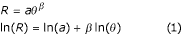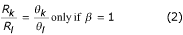### Coefficients of variation

Figure 4 displays comparisons of coefficients of variation found at the probe-level with coefficients of variation found in corresponding gene-expression indexes for the Affymetrix Latin Square dataset. One would expect, based on sampling theory, that the coefficient of variation for the individual data points would be higher than that for an efficient summary statistic by a factor of √J. With typically 16 probes per gene on the HG_U95Av2 array, this ratio would be expected to be 4 in this dataset. Figure 4a shows that the peak (center) contour of CVs (coefficients of variation) is to the right of the dashed line of equality of CV, indicating that the modal CV is slightly lower for MAS5 than the probe level. The peak is above the dotted line of optimal efficiency, suggesting that some of the information at the probe level is not transferred to the index. The median ratio of probe-level CV to MAS5 CV is 0.78, indicating that the MAS5 calculation most often increases CV. Figure 4b shows the modal contour for dChip PM-only nearer to the dotted line of optimal efficiency. The median ratio of probe-level CV to dChip CV is 2.79, which is close to the ideal of 4. Figure 4c shows for MAS5 that the CV results for the TP are similar to the overall results, with modal CV near the line of equality and median CV ratio of 0.74. Figure 4d suggests that dChip CV ratio may be lower for TP, with modal CV nearer to the line of equality and median CV ratio of 1.6. Thus some of the increase in statistical power observed for probe-level analysis may come from inefficient summarization performed with current index methods.

## Discussion

The near certainty of FP results in microarray experiments has fueled continuing demands from reviewers for independent validation of key findings using, for example, reverse transcriptase polymerase chain reaction (RT-PCR) or Northern blot. It has also lead to numerous publications of statistical methods for controlling the false discovery rate [9, 10]. The approach taken here departs from that of methods which start analysis with gene-expression indexes, and instead starts analysis at the probe level.

The central rationale for beginning analysis at the probe level involves consideration of the observation, shown in Figure 4, that CVs for gene-expression indexes across replicate samples do not achieve the level of superiority over those at the probe level that one would expect for an efficient index, according to sampling theory. Thus, it was expected that statistical testing at the probe level would provide more power to accurately ascertain differential expression than testing than testing based on the indexes . This observation on CVs suggests that additional improvements in calculation of gene-expression indexes are possible.

Analysis at the probe level still requires an appropriate normalization of arrays and appropriate statistical methods given the distribution of values. In this analysis, the logit-log transformation was used for normalization; evaluation of the empirical distributions produced by the transformation indicate that the values follow a normal distribution (not shown) and, thus, parametric statistical testing was indicated. The logit transformation is motivated from first-order binding kinetics considered at equilibrium. After performing the logit transformation, values for each array were further mapped to a N(0,1) distribution to ensure comparability between arrays.

The logit transformation has been used successfully for analyzing equilibrium binding of analyte to antibody in radioimmunoassays . The results presented in Figure 2 indicate that use of this with microarrays has been successful. Two parameters, N and A, are fitted to the data for each array. Here, parameter A is assigned the maximum probe intensity +0.1% of the range of intensity values and N the minimum probe intensity -0.1% of the range. This assumes that some probes on the array are saturated and that others are background, which may not be true. One method of ensuring that these assumptions are met and that, therefore, A and N are properly estimated, would be to manipulate some hybridization control spikes to provide a background signal for estimating N and others to provide a saturation signal for estimating A. Ideally, estimation of A and N would be done using many data points.

The combination of logit transformation and probe-level statistical testing provides a means for greatly improving PPV from these experiments with little effect on false negatives. PPV is considered to be a major indicator of performance, based on the intuition that the number of regulated genes is in the region of 100 while the number of unregulated genes is in the region of 10,000. In this dataset there are about 10 'regulated' genes producing a 1000:1 ratio of negatives to positives. At a FP rate of 0.1%, the number of FPs nearly equals the number of known positives. This is an experimentally realistic scenario, although not typical. Furthermore, one would like to know, given a reasonable statistical cut-off, what fraction of the genes in the list might be truly differentially expressed. This is addressed directly by PPV.

Observed reduction in FP with little effect on FN as achieved with Logit-t, compared with testing based on indexes, may result from selecting the median t-score to represent the probe set. Such selection can eliminate the effect on the overall gene score of unresponsive probes or of probes that show large differences due to local artifact. These effects are managed by dChip and MAS5, but perhaps not as well as is achieved with median selection. Using median selection may result in more robust test results.

Irizarry and co-workers recently published the RMA method which they validated using the same Affymetrix Latin Square dataset and one of the Gene Logic datasets. Their ROC results compare very well with those shown here for the RMA, dChip and MAS5 data. They are not exactly the same since Irizarry et al. produced their results from a randomly selected subset of comparisons, while the data presented here are a summary for all comparisons.

The results for the Logit_Exp and Logit_ExpR indexes are intriguing. The observation that Logit_Exp tracked dChip almost identically in the salient region of the ROC curve while Logit_t was much better, suggests that the modeling paradigm may cause the loss of information from the probe-level data. It is not clear if errors are poorly modeled by the minimization scheme or if the model itself has structural problems. One could ask if any probe-level statistical testing procedure can match or improve upon Logit_t. This is an interesting question, but note how simple the Logit_t procedure is: one A (max) and one N (min) per array, followed by the logit-transformation and a Z-transformation. For the Affymetrix Latin Square Dataset, immediate Z-transformation of arrays followed by probe-level testing resulted in 88% sensitivity and 38% PPV, while log-transformation of probe intensities followed by Z-transformation and probe-level testing resulted in 87% sensitivity and 64% PPV for the same data. The logit transformation appears to be useful and the most common modeling assumptions seem to result in significant information loss.

In addition to identifying positive differential expression, one often wants to know the fold changes in an effort to discern major changes from minor ones. Although recent efforts have shown how to produce confidence limits around a fold change for gene-expression indexes , the results presented here suggest that indexes should be adjusted by the β-exponent before performing the ratios and calculating the confidence intervals. All gene-expression indexes have a β-exponent different from 1, and this should be taken into account to improve correspondence between array results and RT-PCR validation.

Other methods for assessing differential expression have been developed. Zhang introduced a method designed for experiments lacking replication which uses the probe-level noise information to estimate a variance used in a pseudo-t-test . Liu et al. presented the algorithm used within the Affymetrix commercial software which compares arrays two at a time . It was not practical to include this here, but the published error rates suggest the results that could be expected. Liu et al. report a FP error rate of 1.26% when optimized with a TP rate of 81% . Extrapolating these rates to the format presented in Table 1, this method could be expected to produce a PPV of 7% and a sensitivity of 75%, comparable to that achieved by the gene-expression index-based methods. Naef et al. have recently published a method useful for detecting differential expression among probe sets near the saturation intensity . Chu et al. reported a general linear modeling approach but did not report on its performance in a setting with known positives and negatives nor in comparison with results using gene-expression indexes . Logit_t is designed to produce high-quality output in the context of typical experiments with replication, therefore special-purpose methods were not included in the comparisons. This work was carried out with C programs or with available software. It was beyond the scope to replicate the work of Chu et al. for inclusion.

## Conclusions

Logit_t can be used to analyze experiments employing Affymetrix arrays and replication and can be expected to produce gene lists having higher PPV than those produced by statistical testing of gene-expression indexes. It seems from this analysis that a gene-expression index that transfers precision of the assay to the index remains elusive. When one appears, it can be expected that statistical test performance for the index will meet or exceed that for probe-level testing by arguments of statistical efficiency. Until then, a combination of probe-level statistical testing and fold change estimation using β-adjusted gene-expression indexes is in order, as is continued reliance on independent validation using RT-PCR or the like.

## Materials and methods

### Data

In the course of developing their most recent statistical algorithm, MAS5, Affymetrix produced and provided data from a set of 59 arrays (HG_U95Av2) organized in a latin-square design . In this dataset, a pool of human samples and cell lines was used to produce a single source of RNA. This was divided into 14 groups comprising 12 groups of three replicates (A-L) and two groups of 12 replicates (group M-P and group Q-T). Each group was spiked with a cocktail containing the specified concentrations of 14 RNAs. It may appear that 14 groups on 14 conditions cannot produce a latin-square. However, since there is only one algorithm for estimating gene expression, the concentration profiles for the 14 spiked genes in this design do produce a latin-square viz-a-viz the MAS5 algorithm or that of any other gene-expression index. This data set not only provides a means to evaluate dose-response for these probe sets, but also provides a means to evaluate the performance of statistical testing procedures. Since a single RNA source was used, any probe set not in the list of 14 should be negative for differential expression. Conversely, all of the probes in the list of 14 should be positive for differential expression. With 14 groups and 14 genes, there are 14 × 13/2 = 91 comparisons and thus 1,274 TP and 1,147,962 true negative incidences of differential gene expression. All analysis was performed on an Apple Xserve Mac OS X 10.2.4. Source code and compilation instructions usable on any Unix system is available via e-mail from the authors.

In addition to the Affymetrix Latin Square dataset, three datasets publicly available from Gene Logic  were also used. These datasets are referred to as Gene Logic Spike, Gene Logic AML and Gene Logic Tonsil. The Gene Logic Spike dataset consists of 26 HG_U95A arrays arranged as follows. All arrays were hybridized with a common complex cRNA derived from acute myeloid leukemia (AML) cell lines. This RNA source was spiked with varying concentrations of sequences complementary to the following 10 control sequences: BioB-5_at, BioB-M_at, BioB-3_at, BioC-5_at, BioC-3_at, BioDn-3_at, DapX-5_at, DapX-M_at, DapX-3_at and CreX-5_at. Spikes were provided at varying concentrations (0 pM, 0.5, 0.75, 1, 1.5, 2, 3, 5, 12.5, 25, 50, 75, 100, 150) and with varying numbers of replicates. This dataset produced 21 usable comparisons or 210 known positives.

The Gene Logic AML dataset comprised 32 HG_U95A arrays each hybridized with the same common RNA source as the Spike dataset with a different arrangement of control spikes. Sequences complementary to the control probes spiked into the Spike dataset were used here along with that for the control probe CreX-3_at. The concentration profiles used are available from Gene Logic; the details are not germane to this report. This dataset provided 55 usable comparisons or 605 known positives.

Finally, the Gene Logic Tonsil dataset comprised 36 HG_U95A arrays hybridized with a common complex RNA produced from pooled tonsil tissue samples. The RNA was spiked with sequences complementary to the same control probes as the AML dataset. The concentrations used and the layout of the concentrations into groups of replicate arrays was slightly different than that for the AML dataset and is available from Gene Logic. This dataset provided 66 usable comparisons or 726 known positives.

### Approach

Affymetrix MAS5, dChip PM-only and RMA gene-expression indexes were obtained using the software provided by the group publishing the index [4, 11]. Affymetrix MAS5 expression indexes were obtained using the Affymetrix commercial software. DChip indexes were obtained using the dChip software available from . RMA indexes were obtained using Bioconductor v1.2 obtained from . Student's t-testing was performed on each probe set on the array for all comparisons within each dataset and the p-values for these procedures retained. No comparisons across datasets were attempted. These results were compared with those of the following novel algorithm.

### Logit-t algorithm

It was reasoned that hybridization to a microarray could be viewed in its dynamics as similar to that of the binding of an analyte to an antibody in a radioimmunoassay . Consider unbound probe, a, and complementary RNA fragment, x, binding to produce a hybrid, y as in a + x ↔ y. The first order kinetic equation is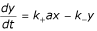which at equilibrium can be expressed as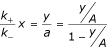where A represents the total amount of probe available for binding. Taking into account an additive non-specific signal, N, we have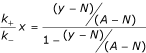which becomes Model (3) upon log-transformation.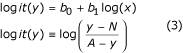Model (3) has two parts: the bottom equation is a transformation requiring no calibration data and is used as the basis for the Logit_t testing procedure; the top equation forms the basis for a gene-expression index and can use calibration data as described below. Parameter A represents maximal signal intensity for the array (saturation) and N represents additive non-specific signal intensity (background) defined as the minimum intensity on the array. There is one A and one N for each array. For each array, A and N were estimated by adding or subtracting 0.1% of the intensity range for the array to the maximum and minimum probe intensities, respectively, found on the array within probe sets. Probe intensities were then logit-transformed using the bottom equation of Model 3 then mapped into N(0,1) by standard Z-transformation. The logit-transformed values before Z-transformation appeared very much like normal distributions and, thus, the Z-transformation is reasonable.

### Probe-level statistical testing: Logit-t

Within a probe set, each PM probe was evaluated across arrays for each of the comparisons using Student's t-tests, and resulting t-values were retained. For a given probe set in a given comparison, Logit-t is defined as the median t-value found among all the perfect match probes in the set. Thresholds for making calls of differential expression or no differential expression were determined by choosing the t-value cutoff corresponding to p < 0.01 for the df of the comparison. For example, when three arrays were compared with three arrays, df = 4 and therefore the t-threshold = 3.7; when three arrays were compared with 12 arrays, df = 13 and therefore the t-threshold = 2.6, and so on.

### Gene-expression indexes: Logit_Expr and Logit_ExprR

To produce a gene-expression index, Model 3 can be re-written as Model 4.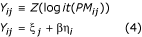The top equation in Model 4 illustrates the logit transformation process described above and results in the PM values transformed into Y values with i indexing the probe set and j indexing the probe within a probe set. Each Y ij value is modeled with a probe-specific intercept, ξ j , and a fixed slope, β, determined from the calibration data shown in Figure 2. The slope is multiplied by the gene-expression index, η i . The intercept, ξ j , can be interpreted as the transformed equilibrium binding constant for the probe and the slope can be considered a transformed exponent that adapts the solution-kinetic equations to the adsorption conditions. Comparing the bottom equation of Model 4 with the top equation of Model 3, ξ j of Model 4 corresponds to b0 of Model 3, β of Model 4 corresponds to b1 of Model 3 and η i of Model 4 corresponds to log(x) of Model 3. It is reasonable to retain the estimate of b1 from Model 3 as the global β since this does not display a strong probe effect. This leaves only ξ j and η i for estimation and relieves the need for auxiliary constraint.

The parameters of interest are η i . Fitting the parameters to the data can be done using various methods. The Logit_Exp index was produced by fitting the parameters to the data using the least-squares equations (5). The Logit_ExpR index, with final R representing robust, was produced by minimizing the sum of squared errors using the median-fitting equations (6).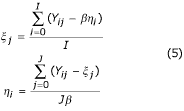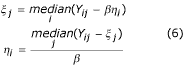### Performance

Statistical performance of each method was evaluated following standard methods. Briefly, a p-value threshold (p < 0.01) and a t-value threshold (based on df) were selected for identifying a positive call of differential expression or a negative call. Using these cutoffs, each probe set in each comparison was labeled differentially expressed or not, and a two-way contingency table produced. With these, the standard performance measures of positive predictive value, negative predictive value and sensitivity, specificity and accuracy were calculated. PPV and sensitivity are reported in Table 1, the other results were uninformative.

### Coefficients of variation

Using available replicates, coefficients of variation

(CV,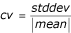)

were computed from the Affymetrix Latin Square dataset for all genes on all groups for MAS5 and dChip PM-only. For probe-level comparison, CVs were calculated for each probe on all groups using the Z-transformed, Logit-transformed probe data. For each probe set and each group, the median CV for all probes in the probe set was selected to represent the set. This yielded one CV for each probe set and each group and each model (MAS5, dChip, probe-level) or 1,148,966 CVs for each model.

## References

1. Chung CH, Bernard PS, Perou CM: Molecular portraits and the family tree of cancer. Nat Genet. 2002, 32: 533-540. 10.1038/ng1038.

2. Holloway AJ, van Laar RK, Tothill RW, Bowtell DD: Options available - from start to finish - for obtaining data from DNA microarrays II. Nat Genet. 2002, 32: 481-489. 10.1038/ng1030.

3. Gu CC, Rao DC, Stormo G, Hicks C, Province MA: Role of gene expression microarray analysis in finding complex disease genes. Genet Epidemiol. 2002, 23: 37-56. 10.1002/gepi.220.

4. Li C, Hung Wong W: Model-based analysis of oligonucleotide arrays: model validation, design issues and standard error application. Genome Biol. 2001, 2: research0032.1-0032.11. 10.1186/gb-2001-2-8-research0032.

5. Lemon WJ, Palatini JJ, Krahe R, Wright FA: Theoretical and experimental comparisons of gene expression indexes for oligonucleotide arrays. Bioinformatics. 2002, 18: 1470-1476. 10.1093/bioinformatics/18.11.1470.

6. Holder D, Raubertas R, Pikounis V, Svetnik V, Soper K: Statistical analysis of high density oligonucleotide arrays: a SAFER approach. In Proceedings of the ASA annual meeting. 2001, Alexandra, VA: American Statistical Association

7. Irizarry RA, Bolstad BM, Collin F, Cope LM, Hobbs B, Speed TO: Summaries of Affymetrix GeneChip probe level data. Nucleic Acids Res. 2003, 31: e15-10.1093/nar/gng015.

8. Affymetrix: Statistical algorithms description document. In Microarray Suite 5. 2002, [http://www.affymetrix.com/support/technical/whitepapers/sadd_whitepaper.pdf]

9. Efron B, Tibshirani R: Empirical bayes methods and false discovery rates for microarrays. Genet Epidemiol. 2002, 23: 70-86. 10.1002/gepi.1124.

10. Sabatti C, Karsten SL, Geschwind DH: Thresholding rules for recovering a sparse signal from microarray experiments. Math Biosci. 2002, 176: 17-34. 10.1016/S0025-5564(01)00102-X.

11. Li C, Wong WH: Model-based analysis of oligonucleotide arrays: expression index computation and outlier detection. Proc Natl Acad Sci USA. 2001, 98: 31-36. 10.1073/pnas.011404098.

12. Lemon WJ, Bernert H, Sun H, Wang Y, You M: Identification of candidate lung cancer susceptibility genes in mouse using oligonucleotide arrays. J Med Genet. 2002, 39: 644-655. 10.1136/jmg.39.9.644.

13. Rodbard D, Lewald JE: Computer analysis of radioligand assay and radioimmunoassay data. Acta Endocrinol Suppl (Copenh). 1970, 147: 79-103.

14. Zhang L, Wang L, Ravindranathan A, Miles MF: A new algorithm for analysis of oligonucleotide arrays: application to expression profiling in mouse brain regions. J Mol Biol. 2002, 317: 225-235. 10.1006/jmbi.2001.5350.

15. Liu WM, Mei R, Di X, Ryder TB, Hubbell E, Dee S, Webster TA, Harrington CA, Ho MH, Baid J, et al: Analysis of high density expression microarrays with signed-rank call algorithms. Bioinformatics. 2002, 18: 1593-1599. 10.1093/bioinformatics/18.12.1593.

16. Naef F, Socci ND, Magnasco M: A study of accuracy and precision in oligonucleotide arrays: extracting more signal at large concentrations. Bioinformatics. 2003, 19: 178-184. 10.1093/bioinformatics/19.2.178.

17. Chu TM, Weir B, Wolfinger R: A systematic statistical linear modeling approach to oligonucleotide array experiments. Math Biosci. 2002, 176: 35-51. 10.1016/S0025-5564(01)00107-9.

18. Affymetrix. [http://www.affymetrix.com/]

19. Gene Logic. [http://qolotus02.genelogic.com/datasets.nsf]

20. dChip Software. [http://www.dchip.org]

21. BioConductor. [http://www.bioconductor.org]

## Acknowledgements

We are grateful to C Charles Gu and Daolong Wang for critical reading of this manuscript and helpful discussions. This work was supported by NIH grants R01CA58554 (M.Y.) & P30CA16058.

## Author information

Authors

### Corresponding author

Correspondence to Ming You.

## Rights and permissions

Reprints and Permissions

Lemon, W.J., Liyanarachchi, S. & You, M. A high performance test of differential gene expression for oligonucleotide arrays. Genome Biol 4, R67 (2003). https://doi.org/10.1186/gb-2003-4-10-r67

• Revised:

• Accepted:

• Published:

• DOI: https://doi.org/10.1186/gb-2003-4-10-r67

### Keywords

• Acute Myeloid Leukemia
• Reverse Transcriptase Polymerase Chain Reaction
• Gene List
• Gene Logic
• Logit Transformation